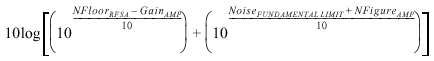# Using CH 1

NI RF Signal Conditioning Devices Help (NI-5690 14.5)

Edition Date: March 2015

Part Number: 371326D-01

»View Product InfoDownload Help (Windows Only)

By switching between the CH 1 main and direct paths as needed, you can customize the NI PXI-5691 application to the needs of your measurement system. Refer to the NI PXI-5691 Block Diagram for an illustration of both paths.

## CH 1 Direct Path

The CH 1 direct path allows you to bypass the step attenuator and amplification in the CH 1 main path. For example, if the level of the signal of interest is within the input range of an NI RF vector signal analyzer, switch to the NI RF amplifier CH 1 direct path. In this configuration, the NI PXI-5691 does not modify the input signal in any way other than a small amount of insertion loss, which can be queried using the ni5690 Get Actual Gain VI. or the ni5690_getActualGain function.

## CH 1 Main Path

The CH 1 main path allows you to apply adjustable gain or attenuation, in nominal 0.5 dB steps, to the signal of interest. For example, if the level of the signal of interest drops below the input range of an NI RF vector signal analyzer, switch to the CH 1 main path and configure for amplification. This path allows you to monitor signals that would normally be invisible to the NI RF vector signal analyzer.

### CH 1 Main Path Considerations

Because the CH 1 main path consists of an adjustable attenuator (automatically controlled by the ni5690 Configure Gain VI or the ni5690_configureGain function) in the amplification path, applying gain at any level other than maximum requires asserting some attenuation. Therefore any CH 1 gain setting, except the maximum, increases the noise figure of the NI PXI-5691 RF amplifier, but consequently improves the linearity.

Whether this increase in noise figure affects your application depends on your system requirements. Use the following equation to determine the noise floor of your system.where    NFloorRFSA is the noise floor of the NI RF signal analyzer in dBm/Hz

GainAMP is the NI PXI-5690 RF amplifier gain setting in dB

NoiseFUNDAMENTAL LIMIT is the fundamental noise floor limit in dBm/Hz (approximately –174 dBm/Hz) at room temperatureNote  –174 dBm/Hz is calculated using this equation: [10 log10(kT) + 30], where k is Boltzmann's constant (1.38 × 10–23), and T is temperature in degrees Kelvin (º C + 273).

NFigureAMP is the noise figure of the NI RF amplifier in dB

Related Topics

Using the NI RF Signal Conditioning Devices With RF Vector Signal Analyzers

Using the NI RF Signal Conditioning Devices With RF Vector Signal Generators